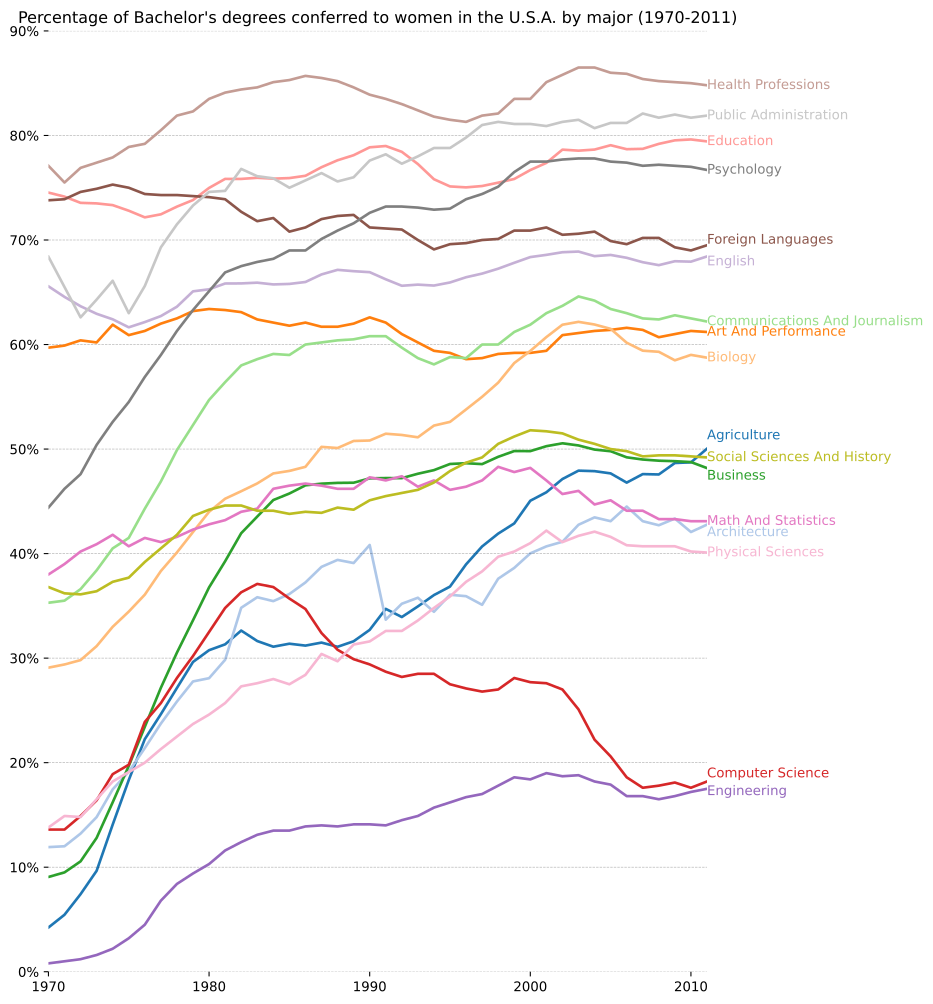# Bachelors Degrees By Gender#

URL: http://matplotlib.org/examples/showcase/bachelors_degrees_by_gender.html

Most examples work across multiple plotting backends equivalent, this example is also available for:

• Bokeh - bachelors_degress_by_gender

```import holoviews as hv
from holoviews import opts
hv.extension('matplotlib')
hv.output(fig='svg')
```## Define data#

```import pandas as pd
from matplotlib.cbook import get_sample_data

fname = get_sample_data('percent_bachelors_degrees_women_usa.csv')

title = ('Percentage of Bachelor\'s degrees conferred to women in '
'the U.S.A. by major (1970-2011)\n')

# These are the colors that will be used in the plot
color_sequence = ['#1f77b4', '#aec7e8', '#ff7f0e', '#ffbb78', '#2ca02c',
'#98df8a', '#d62728', '#ff9896', '#9467bd', '#c5b0d5',
'#8c564b', '#c49c94', '#e377c2', '#f7b6d2', '#7f7f7f',
'#c7c7c7', '#bcbd22', '#dbdb8d', '#17becf', '#9edae5']

# Offsets for degree labels
y_offsets = {'Foreign Languages': 0.5, 'English': -0.5,
'Communications and Journalism': 0.75,
'Art and Performance': -0.25, 'Agriculture': 1.25,
'Social Sciences and History': 0.25, 'Business': -0.75,
'Math and Statistics': 0.75, 'Architecture': -0.75,
'Computer Science': 0.75, 'Engineering': -0.25}

# Load the data into a dataframe and us pd.melt to unpivot the degree column
df = pd.DataFrame(gender_degree_data)
df = pd.melt(df, id_vars='Year', var_name='Degree', value_name='conferred')
df['Degree'] = [d.replace('_', ' ').title() for d in df.Degree]

# Define a formatter that works for both bokeh and matplotlib
def percent_format(x):
try:
return '{:0.0f}%'.format(x)
except:
return '%d%' % x

# Define the value dimensions
vdim = hv.Dimension('conferred', value_format=percent_format, range=(0, 90))

# Define the dataset
ds = hv.Dataset(df, vdims=vdim)
curves = ds.to(hv.Curve, 'Year', groupby='Degree').overlay()

# Define a function to get the text annotations
max_year = ds['Year'].max()
def offset(row):
row['conferred'] += y_offsets.get(row.Degree, 0)
return row
label_df = df[df.Year==max_year].apply(offset, axis=1)
labels = hv.Labels(label_df, ['Year', 'conferred'], 'Degree')
```

## Display in matplotlib#

```# Define a callback to define a custom grid along the y-axis and disabling the (ugly) axis spines
def cb(plot, element):
ax = plot.handles['axis']
ax.grid(True, 'major', 'y', ls='--', lw=.5, c='k', alpha=.3)
ax.spines['bottom'].set_visible(False)
ax.spines['left'].set_visible(False)

(curves * labels).opts(
opts.Curve(
aspect=0.7, bgcolor='white', hooks=[cb], labelled=[], fig_size=350,
show_frame=False, show_grid=False, show_legend=False, xlim=(1970, 2011), xticks=5,
color=hv.Cycle(values=color_sequence), linewidth=2, title=title),
opts.Labels(color='Degree', cmap=color_sequence, horizontalalignment='left'))
```This web page was generated from a Jupyter notebook and not all interactivity will work on this website. Right click to download and run locally for full Python-backed interactivity.